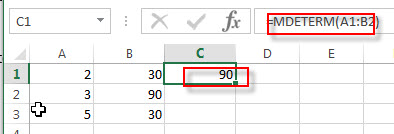# Excel MINVERSE Function

This post will guide you how to use Excel MINVERSE function with syntax and examples in Microsoft excel.

### Description

The Excel MINVERSE function returns the inverse matrix for a given matrix.

The MINVERSE function is a build-in function in Microsoft Excel and it is categorized as a Math and Trigonometry Function.

The MINVERSE function is available in Excel 2016, Excel 2013, Excel 2010, Excel 2007, Excel 2003, Excel XP, Excel 2000, Excel 2011 for Mac.

### Syntax

The syntax of the MINVERSE function is as below:

`=MINVERSE(array)`

Where the MINVERSE function arguments is:

• array -This is a required argument. A numeric array with an equal number of rows and columns. So the array must provide the same number of rows and the same number of columns.

Note:

• The array can be a cell range or an array constant, such as: {2,3;5,6}
• If any cells in array are empty or contain text string, then the MINVERSE function will return the #VALUE! Error.
• If the Array does not have the same number of rows and columns, then the MINVERSE function will return the #VALUE! Error.
• Formulas that return arrays must be entered as array formulas. And if you want to input an array formula, you need to highlight the range of cells that the function is to be entered into, and then press shortcuts Ctrl + Shift + Enter.

Excel MINVERSE Function Examples

The below examples will show you how to use Excel MINVERSE Function to get the inverse matrix for a matrix.

1# to get the inverse matrix for a given matrix A1: C3, enter the following formula in Cell D1.

`=MDETERM(A1:B2)`### Related Functions

• Excel MDETERM function
The Excel MDETERM function returns the matrix determinant of an array. So you can use the MDETERM function to calculate the determinant of a square matrix in Excel.The syntax of the MDETERM function is as below:=MDETERM(array)…
• Excel MMULT function
The Excel MMULT function returns the matrix product of two arrays.If you want to return multiple result in an array on the worksheet, then you need to input an array formula in the MMULT function.The syntax of the MMULT function is as below:=MMULT (array1,array2)…